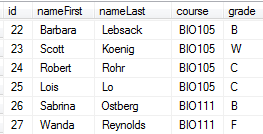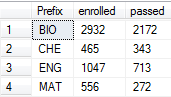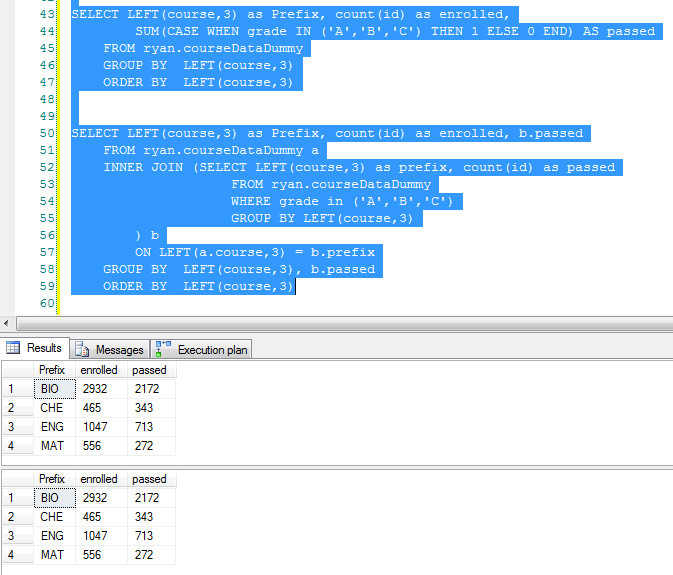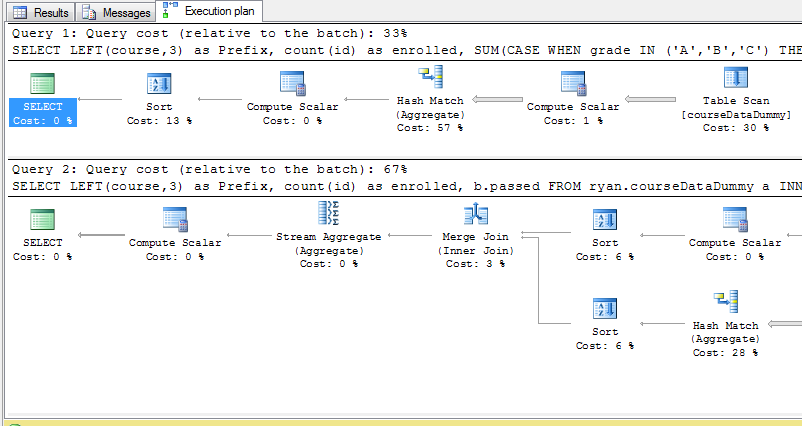My book Mastering PostGIS and OpenStreetMap is available!

# Using SUM and CASE together

By Ryan Lambert -- Published November 18, 2013

Many people are familiar with the `CASE` statement in SQL Server but I do not see examples of using it like I do so commonly for some of my reporting tasks. This post assumes the reader has a working knowledge of CASE statements.

## Getting Started

One question we get in Institutional Research quite frequently is "How many students passed course X". Or some other much more complex variation on the same question. To get started let's assume we have a table that contains one record per student per course they took in a term. It should look something like:For this report we need to get this down to the pass rate by prefix which is the first 3 characters of the course column. Since we need the pass rate for each prefix we will need to know two numbers for the calculations: How many students enrolled in each prefix and how many students passed their courses in each prefix.

My first step is to just get the table down to just the prefix and the number of students enrolled in each prefix. To get the prefix I use the LEFT function to pull out the first 3 characters from the course column. The number enrolled is simply a count of the id.

``````SELECT LEFT(course,3) as Prefix, count(id) as enrolled
GROUP BY  LEFT(course,3)
ORDER BY  LEFT(course,3)
``````

The results:You can see that I only have 4 distinct prefixes in my test data and apparently there are a lot of biology nuts in my random data!

## The Cool Part

Now I need to count the passing grades in my query. The way I typically approach this is to use a CASE statement with SUM like this:

``````SUM(CASE WHEN grade IN ('A','B','C') THEN 1 ELSE 0 END) AS passed
``````

That's a bit intimidating at first, so let's look at just the CASE statement for starters...

``````CASE WHEN grade IN ('A','B','C')
THEN 1
ELSE 0 END
``````

On the first line I'm setting up the condition to find passing grades in the data which we consider to be C or better. If the grade found is in the list of passing grades (A,B, or C) then the record gets assigned "1", otherwise it's assigned "0".

The only other thing is to wrap that case statement inside the SUM() function which sums up all the 1's and 0's to aggregate the data up to match the main query's GROUP BY clause. Let's see it in action. My full query now:

``````SELECT LEFT(course,3) as Prefix, count(id) as enrolled,
SUM(CASE WHEN grade IN ('A','B','C') THEN 1 ELSE 0 END) AS passed
GROUP BY  LEFT(course,3)
ORDER BY  LEFT(course,3)
``````

And the results:I don't know about you, but my next question is how does this perform compared to other methods of achieving the same results? Well, let's find out. The other way I would approach writing this type of query is to setup a sub-query to join in with my original version with the enrolled counts. Here's the query I came up with real quick:

``````SELECT LEFT(course,3) as Prefix, count(id) as enrolled, b.passed
INNER JOIN (SELECT LEFT(course,3) as prefix, count(id) as passed
GROUP BY LEFT(course,3)
) b
ON LEFT(a.course,3) = b.prefix
GROUP BY  LEFT(course,3), b.passed
ORDER BY  LEFT(course,3)
``````

Wow, just looking at the difference I already don't like this version! It's coming out to be 10 lines with my formatting style vs. the 5 lines in my original version. Now to see how they perform, I select both queries and run them at the same time. The top query is the one I like better. The first good news is both methods came out with identical numbers!## Execution Plans:

Below are the execution plans for both queries, one way to compare performance between two queries is to look at the "Query cost (relative to the batch)" that is calculated for each query executed in the batch. Notice that my query with the `SUM(CASE())` is only 33% of the cost, where the other query using the sub query was 67%! Not needing the `JOIN` is a huge savings!## Conclusion

Hopefully someone will find this helpful, I've always enjoyed what can be done with a little ingenuity and the `CASE` statement. I really like seeing that it's more efficient than the next method I would choose when approaching this type of task!

How do you use `CASE` statements in an "outside the box" solution?

By Ryan Lambert
Published November 18, 2013
Last Updated July 12, 2015